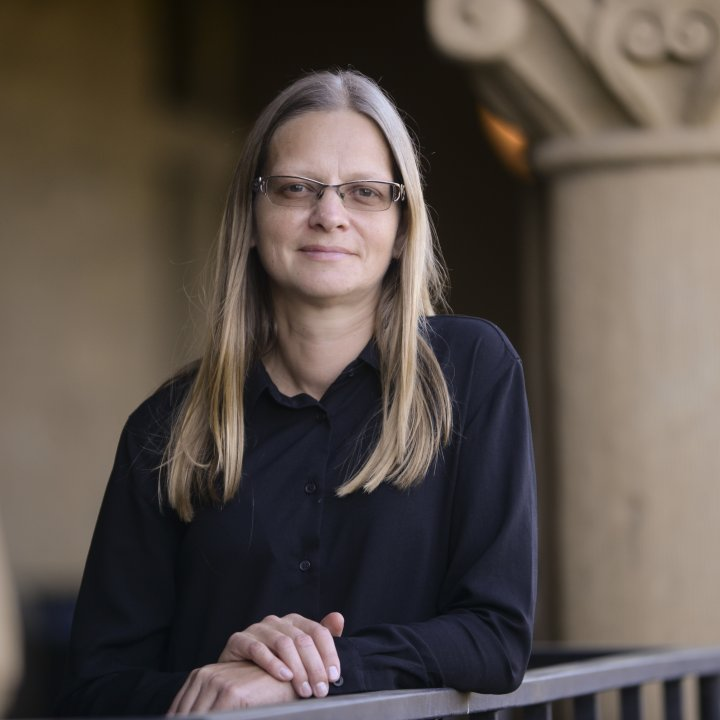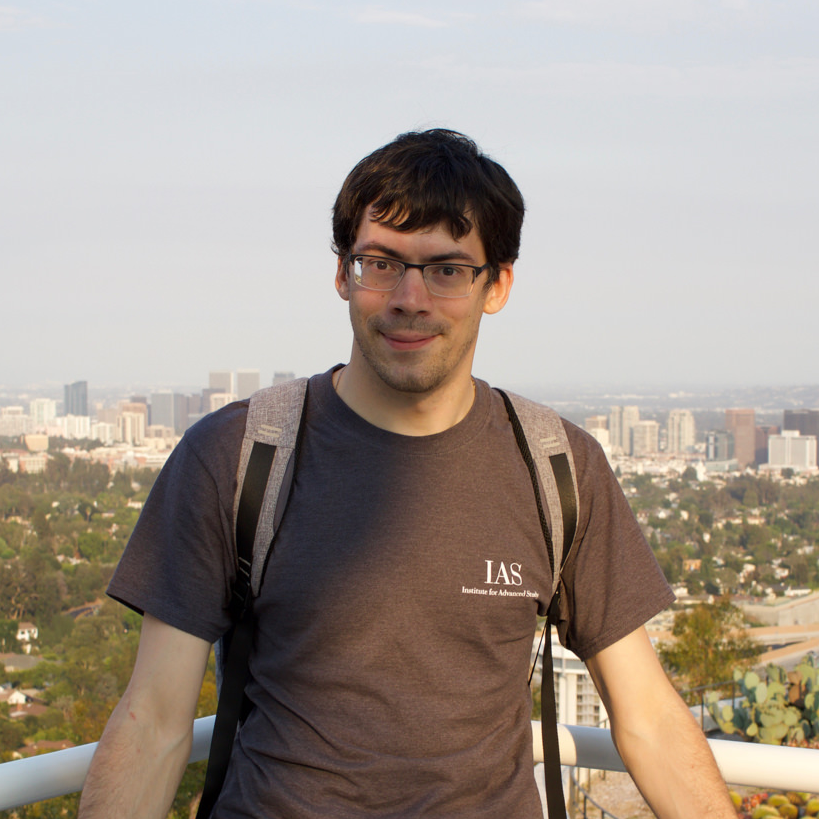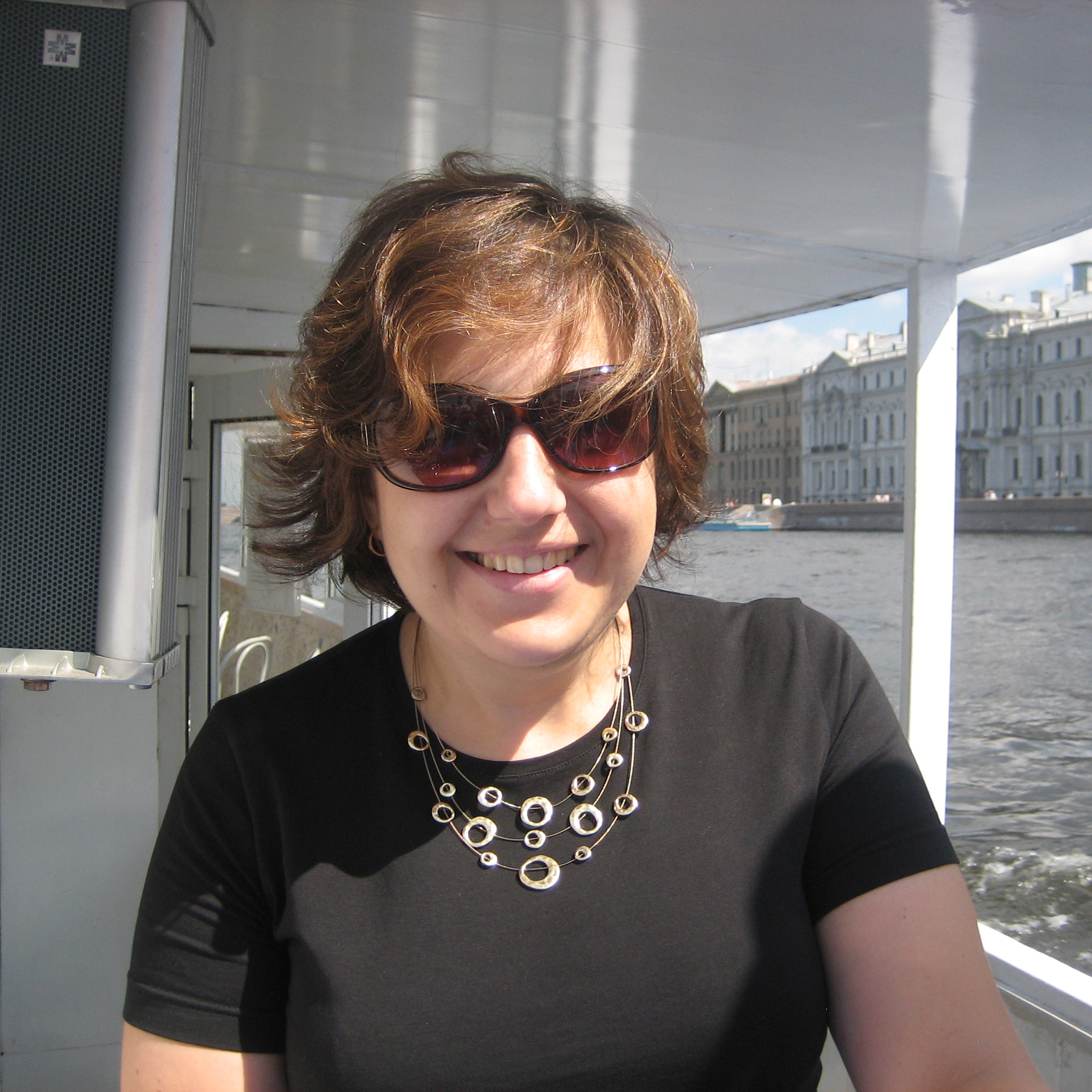# School "Analysis, Probability, Mathematical Physics"

30 November 2020 to 4 December 2020
Online
Europe/Moscow timezone

# ‎Analysis, Probability, Mathematical Physics

### November 30 – December 4, 2020

An advanced school for young researchers featuring three minicourses in vibrant areas of mathematics.  The target audience includes graduate, master and senior bachelor students of any mathematical speciality.

## Lecturers:Eugenia Malinnikova Stanford  UniversityIlya Kachkovskiy Michigan State UniversityTatiana Nagnibeda Université de Genève and SPbSU

## Courses:

### Many forms of the Uncertainty Principle (Eugenia Malinnikova)

There are many mathematical interpretations of the uncertainty principle, which states that the position and momentum of a quantum particle cannot be measured simultaneously or that a signal cannot be well-localized  both in time and in frequency.  In this series of lectures we discuss both the classical statements and their more recent counterparts.

Lecture 1: Heisenberg, Hardy, and Beurling

The classical Heisenberg principle shows that a function and its Fourier transform cannot be localized simultaneously. This mathematical formulation is well-known and follows from the fact that two important operators do not commute. In the first lecture, we discuss the special role played by the Gaussian function in the classical uncertainty relations and formulate a surprising result of Bourgain about a basis consisting of almost perfectly localized functions. We also plan to explain  Beurling's and  Hardy's uncertainty principles.

Lecture 2: Benedicks and Logvinenko-Sereda

The celebrated theorem of Bededicks states that there is no function that together with its Fourier transform is supported by a set of finite measure. We discuss quantitative versions of the result, survey some beautiful inequalities of Fedya Nazarov, and prove the Logvinenko-Sereda theorem on supports of the function and its Fourier transform. The last aim is, if time permits, to formulate a simplified version of the recent results of Bourgain and Dyatlov and Dyatlov and Jin, which are named  the fractal uncertainty principle.

Lecture 3: Slepian-Pollak-Landau

In a remarkable series of works, Slepian, Pollak,  and Landau considered a problem of approximation of a function on a given interval $[-T/2,T/2]$ under the assumption that its frequencies lie in the interval $[-W,W]$. In the1960s, they constructed a basis of prolate spheroidal functions, which has a number of fascinating properties. The aim of this lecture is to present  their main result, the $2WT$ theorem, and review  some further developments on the subject.

Lecture 4: Comments and problems solution

### Localization for quasiperiodic operators with monotone potentials (Ilya Kachkovskiy)

Anderson localization for single-particle quantum systems occurs when the spectra of the corresponding Hamiltonians are purely point and the eigenfunctions decay exponentially. In the physical language, this corresponds to the system being an insulator and was originally discovered by P. Anderson for disordered systems with random potentials. Later, similar phenomena were observed in non-random systems, such as operators with quasiperiodic potentials associated to irrational rotations of the tori. Methods of proof of localization are often quite technical and involve dealing with small denominator problems and KAM resonances. The goal of this course is to study some of these techniques on a large class of examples where the sampling functions for the quasiperiodic potentials are not continuous, but satisfy certain Lipschitz monotonicity properties. For these systems, one can obtain localization in (surprisingly) high generality, but the proofs are less technical, since the resonances have simple structure, or there are no resonances at all. Still, the proofs share many common features with more complicated examples and can serve as an accessible introduction to the subject.

Lecture 1: Overview of one-dimensional methods for ergodic and quasiperiodic operators

In this lecture, we will introduce basic concepts of general spectral theory (the spectral theorem), sufficient conditions for Anderson localization (Schnol-type theorems), and elements of spectral theory of ergodic operators (Lyapunov exponents, integrated density of states, Thouless formula, Kotani theory).

Lecture 2: Large deviation techniques for one-dimensional monotone quasiperiodic operators

In this lecture, we will study the main consequences of monotonicity: estimates for eigenvalue counting functions on boxes and large deviation theorems, both of which are specific for operators with monotone potentials. Afterwards, we will outline the proof of Anderson localization.

Lecture 3: Perturbative methods for multi-dimensional operators with monotone potentials.

The methods for Lectures 1 and 2 are specific for the one-dimensional case. In this lecture, we will discuss several approaches to multi-dimensional problems, based on KAM-type arguments (following Bellissard–Lima–Scoppola) and on direct analysis of Rayleigh–Schrodinger perturbation series. Most of this lecture is independent of Lectures 1 and 2.

Lecture 4: Comments and problems solution

### Probability on graphs and groups (Tatiana Nagnibeda)

Starting with the work of John von Neumann on the Banach-Tarski Paradox, many interesting connections have emerged between the study of infinite (in these lectures, finitely generated) groups and their actions on one hand and probability theory, measure theory and functional analysis on the other hand. In the minicourse we will survey some of these connections, from the more classical ones of expansion and amenability and going on to the more recent study of some models from statistical mechanics on Cayley graphs.

Lecture 1: Spectra of graphs and expanders

We will look at the notion of expansion from geometric and spectral prospectives. We will discuss constructions of infinite families of expanders and the related notions of (non)-amenability and Kazhdan’s Property (T).

Lecture 2: Random walks on groups and graphs

We will start with Kesten’s criterion that characterizes amenable groups in terms of asymptotic behaviour of random walks on their Cayley graphs and will discuss its relevance to the solution of the so-called von Neumann’s Problem. We will also discuss the more recent, ergodic and geometric variations of the problem that, contrary to the classical one, have positive solutions.

Lecture 3: Models of statistical physics on Cayley graphs

We will start with percolation on Cayley graphs and discuss its relation to amenability and other asymptotic properties of the group. Time permitting, we’ll also touch upon some other models, e.g., the uniform spanning forest and the Abelian sandpile model.

Lecture 4: Comments and problems solution

## Institutions participating in the organization of the event:

Starts
Ends
Europe/Moscow
Online
ALL VIDEO RECORDS OF THE SCHOOL
 Berge (Three Surface Theorems) Hedenmalm (Fourier uniqueness in even dimensions) Veprev (Non-existence of a universal zero entropy system for amenable group actions)
 Exercises 1 (Many forms of the Uncertainty Principle) Exercises 2 (Many forms of the Uncertainty Principle) Exercises 3 (Many forms of the Uncertainty Principle) Lecture 1 (Many forms of the Uncertainty Principle) Lecture 2 (Many forms of the Uncertainty Principle) Lecture 3 (Many forms of the Uncertainty Principle)
 Exercises 1 (Probability on graphs and groups) Exercises 2 (Probability on graphs and groups) Exercises 3 (Probability on graphs and groups) Lecture 1 (Probability on graphs and groups) Lecture 2 (Probability on graphs and groups) Lecture 3 (Probability on graphs and groups) Lecture 4 (Probability on graphs and groups) Literature "Eigenvalues of graphs and a simple proof of a theorem of Greenberg" (Probability on graphs and groups) Literature "On the second eigenvalue of a graph" (Probability on graphs and groups)
 Exercises 1,2,3 (Quasiperiodic operators with monotone potentials) Lecture 1 (Localization for quasiperiodic operators with monotone potentials) Lecture 2 (Localization for quasiperiodic operators with monotone potentials) Lecture 3 (Localization for quasiperiodic operators with monotone potentials) Literature (Quasiperiodic operators with monotone potentials)